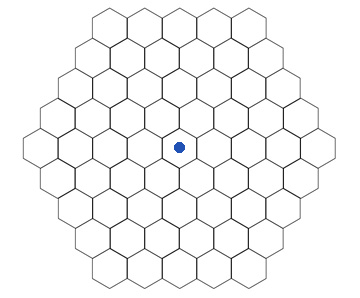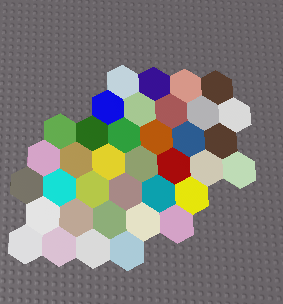# Hexagonal grid generation

I am wanting to create a hexagon grid for a game I am working on, however I can’t seem to get the offset done correctly. I have looked around the forum for a bit and on other websites, but I can’t find a solution for the shape I want. This is the desired shape:This is the shape I’m getting:Here is my current code, If I am missing anything or if you have any unrelated way to improve my code I would love the input, thank you!

``````local replicatedStorage = game:GetService("ReplicatedStorage")
local tile = replicatedStorage:WaitForChild("Tile")

local boardSize = 7
local pos = Vector3.new(0,0,0)

for x=1,boardSize do
local start,finish = nil,nil
if x<math.ceil(boardSize/2) then
start,finish = math.ceil(boardSize/2)-x+1,boardSize
else
start,finish = 1,boardSize-math.floor(x-boardSize/2)
end
for y=start,finish do
local newTile = tile:Clone()
newTile.Parent = workspace
local offset = 0
if y%2==0 then
offset = newTile.Size.X/2
end
newTile.Position = pos + Vector3.new(x*newTile.Size.X+offset,0,y*newTile.Size.Z*0.75)
newTile.BrickColor = BrickColor.Random()
end
end
``````
1 Like

Found a solution:
Because each 2nd row is offset by 1 tile, I am able to add math.floor(y/2) to x in order to counterbalance that offset.

``````local replicatedStorage = game:GetService("ReplicatedStorage")
local tile = replicatedStorage:WaitForChild("Tile")

local boardSize = 7
local pos = Vector3.new(0,0,0)

for x=1,boardSize do
local start,finish = nil,nil
if x<math.ceil(boardSize/2) then
start,finish = math.ceil(boardSize/2)-x+1,boardSize
else
start,finish = 1,boardSize-math.floor(x-boardSize/2)
end
for y=start,finish do
local newTile = tile:Clone()
newTile.Parent = workspace
local offset = 0
if y%2==0 then
offset = newTile.Size.X*0.5
end
newTile.Position = pos + Vector3.new((x+math.floor(y/2))*newTile.Size.X-offset,0,y*newTile.Size.Z*0.75)
newTile.BrickColor = BrickColor.Random()
end
end
``````
3 Likes